UPSC  >  Arun Sharma Test: HCF & LCM

# Arun Sharma Test: HCF & LCM - UPSC

Test Description

## 10 Questions MCQ Test CSAT Preparation - Arun Sharma Test: HCF & LCM

Arun Sharma Test: HCF & LCM for UPSC 2023 is part of CSAT Preparation preparation. The Arun Sharma Test: HCF & LCM questions and answers have been prepared according to the UPSC exam syllabus.The Arun Sharma Test: HCF & LCM MCQs are made for UPSC 2023 Exam. Find important definitions, questions, notes, meanings, examples, exercises, MCQs and online tests for Arun Sharma Test: HCF & LCM below.
Solutions of Arun Sharma Test: HCF & LCM questions in English are available as part of our CSAT Preparation for UPSC & Arun Sharma Test: HCF & LCM solutions in Hindi for CSAT Preparation course. Download more important topics, notes, lectures and mock test series for UPSC Exam by signing up for free. Attempt Arun Sharma Test: HCF & LCM | 10 questions in 10 minutes | Mock test for UPSC preparation | Free important questions MCQ to study CSAT Preparation for UPSC Exam | Download free PDF with solutions
 1 Crore+ students have signed up on EduRev. Have you?
Arun Sharma Test: HCF & LCM - Question 1

### Find the lowest common multiple of 24, 36 and 40.

Detailed Solution for Arun Sharma Test: HCF & LCM - Question 1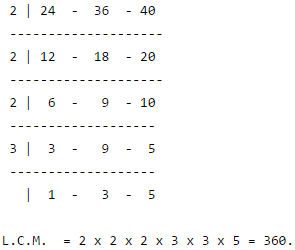Arun Sharma Test: HCF & LCM - Question 2

### The least number which should be added to 2497 so that the sum is exactly divisible by 5, 6, 4 and 3 is:

Detailed Solution for Arun Sharma Test: HCF & LCM - Question 2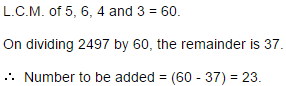Arun Sharma Test: HCF & LCM - Question 3

###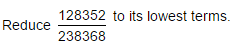Detailed Solution for Arun Sharma Test: HCF & LCM - Question 3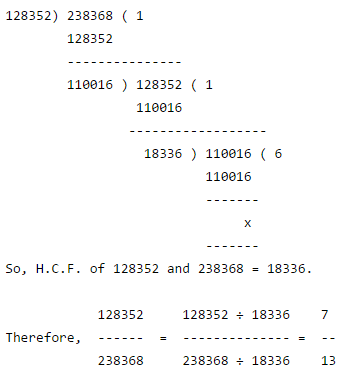Arun Sharma Test: HCF & LCM - Question 4

The least number which when divided by 5, 6 , 7 and 8 leaves a remainder 3, but when divided by 9 leaves no remainder, is:

Detailed Solution for Arun Sharma Test: HCF & LCM - Question 4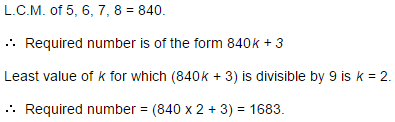Arun Sharma Test: HCF & LCM - Question 5

A, B and C start at the same time in the same direction to run around a circular stadium. A completes a round in 252 seconds, B in 308 seconds and c in 198 seconds, all starting at the same point. After what time will they again at the starting point ?

Detailed Solution for Arun Sharma Test: HCF & LCM - Question 5

L.C.M. of 252, 308 and 198 = 2772.

So, A, B and C will again meet at the starting point in 2772 sec. i.e., 46 min. 12 sec.

Arun Sharma Test: HCF & LCM - Question 6

The H.C.F. of two numbers is 11 and their L.C.M. is 7700. If one of the numbers is 275, then the other is:

Detailed Solution for Arun Sharma Test: HCF & LCM - Question 6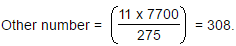Arun Sharma Test: HCF & LCM - Question 7

What will be the least number which when doubled will be exactly divisible by 12, 18, 21 and 30 ?

Detailed Solution for Arun Sharma Test: HCF & LCM - Question 7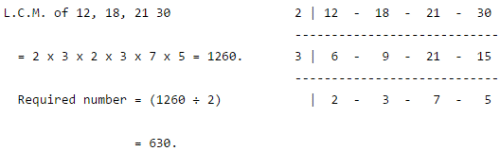Arun Sharma Test: HCF & LCM - Question 8

The ratio of two numbers is 3 : 4 and their H.C.F. is 4. Their L.C.M. is:

Detailed Solution for Arun Sharma Test: HCF & LCM - Question 8

Let the numbers be 3x and 4x. Then, their H.C.F. = x. So, x = 4.

So, the numbers 12 and 16.

L.C.M. of 12 and 16 = 48.

Arun Sharma Test: HCF & LCM - Question 9

The smallest number which when diminished by 7, is divisible 12, 16, 18, 21 and 28 is:

Detailed Solution for Arun Sharma Test: HCF & LCM - Question 9

Required number = (L.C.M. of 12,16, 18, 21, 28) + 7

= 1008 + 7

= 1015

Arun Sharma Test: HCF & LCM - Question 10

252 can be expressed as a product of primes as:

Detailed Solution for Arun Sharma Test: HCF & LCM - Question 10

Clearly, 252 = 2 x 2 x 3 x 3 x 7.

## CSAT Preparation

197 videos|151 docs|200 tests
Information about Arun Sharma Test: HCF & LCM Page
In this test you can find the Exam questions for Arun Sharma Test: HCF & LCM solved & explained in the simplest way possible. Besides giving Questions and answers for Arun Sharma Test: HCF & LCM, EduRev gives you an ample number of Online tests for practice

## CSAT Preparation

197 videos|151 docs|200 tests

### How to Prepare for UPSC

Read our guide to prepare for UPSC which is created by Toppers & the best Teachers